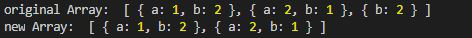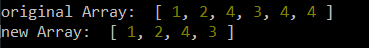GFG App
Open AppBrowser
Continue

# Lodash _.dropRightWhile() Function

Lodash is a JavaScript library that works on the top of underscore.js. Lodash helps in working with arrays, strings, objects, numbers, etc.
The _.dropRightWhile() function is used to delete the slice of the array excluding elements dropped from the end until the predicate function returns false.

Syntax:

`_.dropRightWhile(array, [predicate=_.identity])`

Parameter:

• array: It is the array from which the elements are to be removed.
• predicate: It is the function that iterates over each element of the array and returns true or false on the basis of the condition given.

Return: It returns the array.

Note: Install the lodash module by using the command `npm install lodash` before using the code given below.

Example 1:

 `// Requiring the lodash library ` `const _ = require(``"lodash"``); ` ` `  `// Original array ` `let array1 = [ ` `    ``{ ``"a"``: 1, ``"b"``: 2 }, ` `    ``{ ``"a"``: 2, ``"b"``: 1 },  ` `    ``{ ``"b"``: 2 } ` `] ` ` `  `// Using _.dropRightWhile() function ` `let newArray = _.dropRightWhile(array1, (e) => { ` `    ``return` `e.b == 2; ` `}); ` ` `  `// Original Array ` `console.log(``"original Array: "``, array1) ` ` `  `// Printing the newArray ` `console.log(``"new Array: "``, newArray) `

Output:Example 2: In this example, the predicate function returns false it does not check further and returns the sliced array. Please note that it is different from drop while as it drops element from the right side and not left.

 `// Requiring the lodash library ` `const _ = require(``"lodash"``); ` ` `  `// Original array ` `let array1 = [1, 2, 4, 3, 4, 4] ` ` `  `// Using _.dropRightWhile() function ` `let newArray = _.dropRightWhile(array1, (e) => { ` `    ``return` `e == 4; ` `}); ` ` `  `// Original Array ` `console.log(``"original Array: "``, array1) ` ` `  `// Printing the newArray ` `console.log(``"new Array: "``, newArray) `

Output:My Personal Notes arrow_drop_up